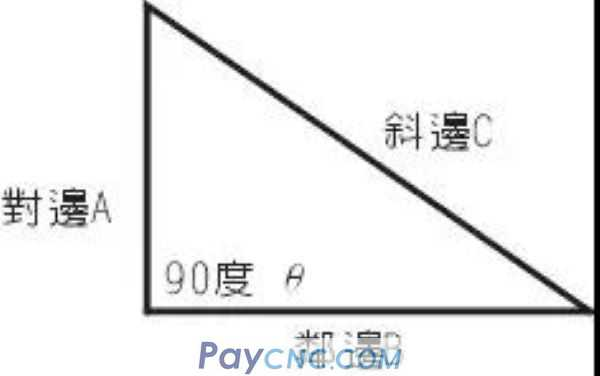Products Catalogue Home     |     About Us    |     Retrofit     |     Download     |     News     |     Tech Support     |     Contact Us     |
ppr fittings-NF-4011-Newsun Industry Co., Ltd
 Home > Tech Support >

## Trigonometric function for CNCAngle function relationship (Sine) Sin θ = Opposite side A / Hypotenuse C (Cosine) Cosθ = adjacent side B / hypotenuse C (Tangent) Tanθ = Opposite side A / Adjacent side B   Opposite side A = Hypotenuse C * Sinθ Opposite side A = Adjacent side B * Tanθ Neighbor B = Hypotenuse C * Cosθ Neighbor B = Opposite side A / Tanθ Hypotenuse C = Opposite side A / Sinθ Hypotenuse C = Adjacent side B / Cosθ Example: It is known that the hypotenuse C=20, Angle θ=35 degrees, find the opposite side A and the adjacent side B   　　　 Opposite side A = Hypotenuse C * Sinθ = 20 * Sin (35) = 20 * 0.573576 = 11.471   　　　Neighbor B = Hypotenuse C * Cosθ = 20 * Cos (35) = 20 * 0.81915 = 16.383 The relationship between taper of general lathe and trigonometric function 　 Taper ratio T=(large diameter D-small diameter d) / (Length L) Tanθ= (Large diameter D-small diameter d) / (2*length L) D= d + 2*L* Tanθ d= D-2*L* Tanθ θ= Tan-((D-d) / 2L)

—[Close]— —[ Back]— —[ Print]—